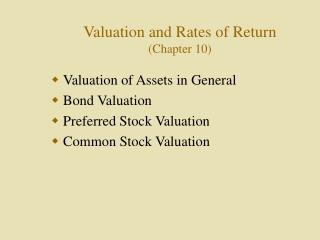DownloadDownload PresentationValuation and Rates of Return (Chapter 10)

# Valuation and Rates of Return (Chapter 10)

Download Presentation## Valuation and Rates of Return (Chapter 10)

- - - - - - - - - - - - - - - - - - - - - - - - - - - E N D - - - - - - - - - - - - - - - - - - - - - - - - - - -
##### Presentation Transcript

1. Valuation and Rates of Return(Chapter 10) • Valuation of Assets in General • Bond Valuation • Preferred Stock Valuation • Common Stock Valuation

2. Valuation of Assets in General • The following applies to any financial asset: V = Current value of the asset Ct = Expected future cash flow in period (t) k = Investor’s required rate of return Note: When analyzing various assets (e.g., bonds, stocks), the formula below is simply modified to fit the particular kind of asset being evaluated.

3. Valuation of Assets (Continued) • Determining Intrinsic Value: • The intrinsic value of an asset (the perceived value by an individual investor) is determined by discounting all of the future cash flows back to the present at the investor’s required rate of return (i.e., Given the Ct’s and k, calculate V). • Determining Expected Rate of Return: • Find that rate of discount at which the present value of all future cash flows is exactly equal to the current market value. (i.e., Given the Ct’s and V, calculate k).

4. Investors’ Required Rates of Return(Nominal Risk-Free Rate Plus a Risk Premium) Required Return Risk

5. Bond Valuation Pb = Price of the bond It = Interest payment in period (t) (Coupon interest) Pn = Principal payment at maturity (par value) Y = Bondholders’ required rate of return or yield to maturity Annual Discounting:

6. Bond Valuation (Continued) • Semiannual Discounting: • Divide the annual interest payment by 2 • Divide the annual required rate of return by 2 • Multiply the number of years by 2

7. Determining Intrinsic Value • The investor’s perceived value • Given It, Pn, and Y, solve for Pb • Determining Yield to Maturity • Expected rate of return • Given It, Pn, and Pb, solve for Y

8. Calculating Yield to Maturity • Trial and Error: Keep guessing until you find the rate whereby the present value of the interest and principal payments is equal to the current price of the bond. (necessary procedure without a financial calculator or computer). • Easiest Approach: Use a computer or financial calculator. Note, however, that it is extremely important to understand the mechanics that go into the calculations.

9. Relationship Between Interest Rates, Time to Maturity, and Bond Prices • For both bonds shown below, the coupon rate is 10% (i.e., It = \$100 and Pn = \$1,000). Bond Price 5 year bond 20 year bond Yield to Maturity (Y) - Percent

10. Relationship Between Coupon Rate and Yield to Maturity (Y) or Current Interest Rates • 1: When Y = coupon rate, Pb = Pn • 2. When Y < coupon rate, Pb >Pn • (Bond sells at a premium) • 3. When Y > coupon rate, Pb < Pn • (Bond sells at a discount) Also Note: If interest rates (Y) go up, bond prices drop, and vice versa. Furthermore, the longer the maturity of the bond, the greater the price change for any given change in interest rates.

11. Preferred Stock Valuation • Ordinary preferred stock usually represents a perpetuity (a stream of equal dividend payments expected to continue forever). • Pp = Price of the preferred stock Dp = Annual dividend (a constant amount) kp = Required rate of return • Determining Intrinsic Value:

12. Preferred Stock (Continued) • Algebraic proof that Equation 1 is equal to Equation 2 on the previous slide when the dividend is a constant amount can be found in many finance texts. • Determining Expected Rate of Return:

13. Common Stock Valuation

14. Common Stock Valuation Continued

15. Constant Growth Rate Model

16. Valuing Common StockUsing Valuation Ratios • Price Per Share = (EPS)(P/E) • Price Per Share = (BV Per Share)(Price/Book) • Price Per Share = (Sales Per Share)(Price/Sales)